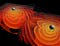Black holes may be our best option for exploring quantum gravitational effects, as the space very close to the central singularity is where those effects are expected to be most important. However, below a certain distance scale, we are unable to accurately describe the Universe, even in theory. The existence of a smallest distance scale at which the laws of physics presently makes sense is a puzzle yet-to-be-solved for physicists. (NASA/AMES RESEARCH CENTER/C. HENZE)

# What Is The Smallest Possible Distance In The Universe?

## The Planck length is a lot smaller than anything we’ve ever accessed. But is it a true limit?

If you wanted to understand how our Universe operates, you’d have to examine it at a fundamental level. Macroscopic objects are made up of particles, which can only themselves be detected by going to subatomic scales. To examine the Universe’s properties, you must to look at the smallest constituents on the smallest possible scales. Only by understanding how they behave at this fundamental level can we hope to understand how they join together to create the human-scale Universe we’re familiar with.

But you can’t extrapolate what we know about even the small-scale Universe to arbitrarily small distance scales. If we decide to go down to below about 10^-35 meters ⁠ — the Planck distance scale ⁠ — our conventional laws of physics only give nonsense for answers. Here’s the story of why, below a certain length scale, we cannot say anything physically meaningful.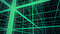We often visualize space as a 3D grid, even though this is a frame-dependent oversimplification when we consider the concept of spacetime. The question of whether space and time are discrete or continuous, and whether there’s a smallest possible length scale, is still unanswered. However, we do know that below the Planck distance scale, we cannot predict anything with any accuracy at all. (REUNMEDIA / STORYBLOCKS)

Imagine, if you like, one of the classic problems of quantum physics: the particle-in-a-box. Imagine any particle you like, and imagine that it’s somehow confined to a certain small volume of space. Now, in this quantum game of peek-a-boo, we’re going to ask the most straightforward question you can imagine: “where is this particle?”

You can make a measurement to determine the particle’s position, and that measurement will give you an answer. But there will be an inherent uncertainty associated with that measurement, where the uncertainty is caused by the quantum effects of nature.

How large is that uncertainty? It’s related to both ħ and L, where ħ is Planck’s constant and L is the size of the box.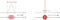This diagram illustrates the inherent uncertainty relation between position and momentum. When one is known more accurately, the other is inherently less able to be known accurately. (WIKIMEDIA COMMONS USER MASCHEN)

For most of the experiments we perform, Planck’s constant is small compared to any actual distance scale we’re capable of probing, and so when we examine the uncertainty we get — related to both ħ and L — we’ll see a small inherent uncertainty.

But what if L is small? What if L is so small that, relative to ħ, it’s either comparably sized or even smaller?

This is where you can see the problem start to arise. These quantum corrections that occur in nature don’t simply arise because there’s the main, classical effect, and then there are quantum corrections of order ~ħ that arise. There are corrections of all orders: ~ħ, ~ħ², ~ħ³, and so on. There’s a certain length scale, known as the Planck length, where if you reach it, the higher-order terms (which we usually ignore) become just as important as, or even more important than, the quantum corrections we normally apply.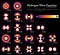The energy levels and electron wavefunctions that correspond to different states within a hydrogen atom, although the configurations are extremely similar for all atoms. The energy levels are quantized in multiples of Planck’s constant, but the sizes of the orbitals and atoms are determined by the ground-state energy and the electron’s mass. Additional effects may be subtle, but shift the energy levels in measurable, quantifiable fashions. Note that the potential created by the nucleus acts like a ‘box’ that confines the electron’s physical extent, similar to the particle-in-a-box thought experiment. (POORLENO OF WIKIMEDIA COMMONS)

What is that critical length scale, then? The Planck scale was first put forth by physicist Max Planck more than 100 years ago. Planck took the three constants of nature:

1. G, the gravitational constant of Newton’s and Einstein’s theories of gravity,
2. ħ, Planck’s constant, or the fundamental quantum constant of nature, and
3. c, the speed of light in a vacuum,

and realized that you could combine them in different ways to get a single value for mass, another value for time, and another value for distance. These three quantities are known as the Planck mass (which comes out to about 22 micrograms), the Planck time (around 10^-43 seconds), and the Planck length (about 10^-35 meters). If you put a particle in a box that’s the Planck length or smaller, the uncertainty in its position becomes greater than the size of the box.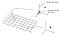If you confine a particle to a space, and try to measure its properties, there will be quantum effects proportional to Planck’s constant and the size of the box. If the box is very small, below a certain length scale, these properties become impossible to calculate. (ANDY NGUYEN / UT-MEDICAL SCHOOL AT HOUSTON)

But there’s a lot more to the story than that. Imagine you had a particle of a certain mass. If you compressed that mass down into a small enough volume, you’d get a black hole, just like you would for any mass. If you took the Planck mass — which is defined by the combination of those three constants in the form of √(ħc/G) — and asked that question, what sort of answer would you get?

You’d find that the volume of space you needed that mass to occupy would be a sphere whose Schwarzschild radius is double the Planck length. If you asked how long it would take to cross from one end of the black hole to the other, the length of time is four times the Planck time. It’s no coincidence that these quantities are related; that’s unsurprising. But what might be surprising is what it implies when you start asking questions about the Universe at those tiny distance and time scales.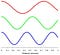The energy of a photon depends on the wavelength it has; longer wavelength are lower in energy and shorter wavelengths are higher. In principle, there is no limit to how short a wavelength can be, but there are other physics concerns that cannot be ignored. (WIKIMEDIA COMMONS USER MAXHURTZ)

In order to measure anything at the Planck scale, you’d need a particle with sufficiently high energy to probe it. The energy of a particle corresponds to a wavelength (either a photon wavelength for light or a de Broglie wavelength for matter), and to get down to Planck lengths, you need a particle at the Planck energy: ~10¹⁹ GeV, or approximately a quadrillion times greater than the maximum LHC energy.

If you had a particle that actually achieved that energy, its momentum would be so large that the energy-momentum uncertainty would render that particle indistinguishable from a black hole. This is truly the scale at which our laws of physics break down.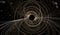The simulated decay of a black hole not only results in the emission of radiation, but the decay of the central orbiting mass that keeps most objects stable. Black holes are not static objects, but rather change over time. For the lowest-mass black holes, evaporation happens the fastest. (EU’S COMMUNICATE SCIENCE)

When you examine the situation in greater detail, it only gets worse. If you start thinking about quantum fluctuations inherent to space (or spacetime) itself, you’ll recall there’s also an energy-time uncertainty relation. The smaller the distance scale, the smaller the corresponding timescale, which implies a larger energy uncertainty.

At the Planck distance scale, this implies the appearance of black holes and quantum-scale wormholes, which we cannot investigate. If you performed higher-energy collisions, you’d simply create larger mass (and larger size) black holes, which would then evaporate via Hawking radiation.An illustration of the concept of quantum foam, where quantum fluctuations are large, varied, and important on the smallest of scales. The energy inherent to space fluctuates in large amounts on these scales. If you view scales that are small enough, such as approaching the Planck scale, the fluctuations become large enough that they create black holes spontaneously. (NASA/CXC/M.WEISS)

You might argue that, perhaps, this is why we need quantum gravity. That when you take the quantum rules we know and apply them to the law of gravity we know, this is simply highlighting a fundamental incompatibility between quantum physics and General Relativity. But it’s not so simple.

Energy is energy, and we know it causes space to curve. If you start attempting to perform quantum field theory calculations at or near the Planck scale, you no longer know what type of spacetime to perform your calculations in. Even in quantum electrodynamics or quantum chromodynamics, we can treat the background spacetime where these particles exist to be flat. Even around a black hole, we can use a known spatial geometry. But at these ultra-intense energy, the curvature of space is unknown. We cannot calculate anything meaningful.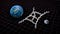Quantum gravity tries to combine Einstein’s General theory of Relativity with quantum mechanics. Quantum corrections to classical gravity are visualized as loop diagrams, as the one shown here in white. Whether space (or time) itself is discrete or continuous is not yet decided, as is the question of whether gravity is quantized at all, or particles, as we know them today, are fundamental or not. But if we hope for a fundamental theory of everything, it must include quantized fields. (SLAC NATIONAL ACCELERATOR LAB)

At energies that are sufficiently high, or (equivalently) at sufficiently small distances or short times, our current laws of physics break down. The background curvature of space that we use to perform quantum calculations is unreliable, and the uncertainty relation ensures that our uncertainty is larger in magnitude than any prediction we can make. The physics that we know can no longer be applied, and that’s what we mean when we say that “the laws of physics break down.”

But there might be a way out of this conundrum. There’s an idea that’s been floating around for a long time — since Heisenberg, actually — that could provide a solution: perhaps there’s a fundamentally minimal length scale to space itself.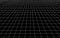A representation of flat, empty space with no matter, energy or curvature of any type. If this space is fundamentally discrete, meaning there’s a minimum length scale to the Universe, we should be able to design an experiment that, at least in theory, shows that behavior. (AMBER STUVER, FROM HER BLOG, LIVING LIGO)

Of course, a finite, minimum length scale would create its own set of problems. In Einstein’s theory of relativity, you can put down an imaginary ruler, anywhere, and it will appear to shorten based on the speed at which you move relative to it. If space were discrete and had a minimum length scale, different observers — i.e., people moving at different velocities — would now measure a different fundamental length scale from one another!

That strongly suggests there would be a “privileged” frame of reference, where one particular velocity through space would have the maximum possible length, while all others would be shorter. This implies that something that we currently think is fundamental, like Lorentz invariance or locality, must be wrong. Similarly, discretized time poses big problems for General Relativity.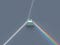This illustration, of light passing through a dispersive prism and separating into clearly defined colors, is what happens when many medium-to-high energy photons strike a crystal. If we were to set this up with just a single photon, the amount the crystal moved could be in a discrete number of spatial ‘steps.’ (WIKIMEDIA COMMONS USER SPIGGET)

Still, there may actually be a way to test whether there is a smallest length scale or not. Three years before he died, physicist Jacob Bekenstein put forth a brilliant idea for an experiment. If you pass a single photon through a crystal, you’ll cause it to move by a slight amount.

Because photons can be tuned in energy (continuously) and crystals can be very massive compared to a photon’s momentum, we could detect whether the crystal moves in discrete “steps” or continuously. With low-enough energy photons, if space is quantized, the crystal would either move a single quantum step or not at all.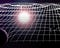The fabric of spacetime, illustrated, with ripples and deformations due to mass. However, even though there are many things happening in this space, it does not need to be broken up into individual quanta itself.(EUROPEAN GRAVITATIONAL OBSERVATORY, LIONEL BRET/EUROLIOS)

At present, there is no way to predict what’s going to happen on distance scales that are smaller than about 10^-35 meters, nor on timescales that are smaller than about 10^-43 seconds. These values are set by the fundamental constants that govern our Universe. In the context of General Relativity and quantum physics, we can go no farther than these limits without getting nonsense out of our equations in return for our troubles.

It may yet be the case that a quantum theory of gravity will reveal properties of our Universe beyond these limits, or that some fundamental paradigm shifts concerning the nature of space and time could show us a new path forward. If we base our calculations on what we know today, however, there’s no way to go below the Planck scale in terms of distance or time. There may be a revolution coming on this front, but the signposts have yet to show us where it will occur.

Starts With A Bang is now on Forbes, and republished on Medium thanks to our Patreon supporters. Ethan has authored two books, Beyond The Galaxy, andTreknology: The Science of Star Trek from Tricorders to Warp Drive.

Written by

## Starts With A Bang!

#### The Universe is out there, waiting for you to discover it.

Welcome to a place where words matter. On Medium, smart voices and original ideas take center stage - with no ads in sight. Watch
Follow all the topics you care about, and we’ll deliver the best stories for you to your homepage and inbox. Explore
Get unlimited access to the best stories on Medium — and support writers while you’re at it. Just \$5/month. Upgrade